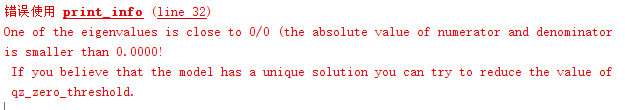# Exogenous impact problem

Excuse me, I have a problem. Can you help me? Thank you very much

When exogenous shocks are not first-order moments, but include second-order moments, how to code them in Dynare

What do you mean? An uncertainty shock?

First of all, thank you for your reply. Yes, I am referring to an uncertain shock, such as the introduction of an uncertain shock into a total factor productivity shock, and the uncertain shock is a random wave function. Under normal circumstances, epsilon of exogenous shock obeys normal distribution of zero mean. For example, it is expressed as
“Varexo E; Shocks. Var e; Stderr 0.01; End.”
In this case, epsilon ~NID (0, var (ε))

However, when epsilon ~IID^+ (E (ε), Var (ε)) (in this case, there are first and second order moments under this probability distribution), how to express in the code?

All stochastic shocks in Dynare are mean 0. You can always normalize them that way. Regarding uncertainty shocks, see e.g. DSGE_mod/Basu_Bundick_2017 at master · JohannesPfeifer/DSGE_mod · GitHub

thank you for your reply，that helped me a lot.May I ask which article this program comes from？

Basu/Bundick (2017): “Uncertainty shocks in a model of effective demand”, Econometrica, 85(3), pp. 937-958

All right, thank you very much

Hi,can you tell me the cause of mistake “The Jacobian matrix evaluated at the steady state contains elements that are not real or
are infinite.”

Usually, it happens if some steady state becomes 0 or negative and that variable is raised to a fractional power.

I’m sorry to bother you again,I still haven’t solved my problem, and the code in the mod file I refer to in this article doesn’t work. I would like to ask if there is any code for reference in the case of binary matrix in the model.

1. Without the file it is impossible to tell what is going on.
2. What do you mean with ?

Sorry, I didn’t state clear, I mean the model has a second order matrix.How to deal with it?

Can you provide a specific example?

monetary policy and natural disasters in a dsge model：dsge模型中的货币政策和自然灾害 - 豆丁网 (docin.com)The article I referred to was this one“monetary policy and natural disasters in a dsge model”by Benjamin D. Keen* and Michael R. Pakkoj. In this article,they use a two-state Markov switching process to define whether a disaster has occurred.Can you open this website and picture, I’m curious to know, how to handle this part in Dynare?

To me this looks as if there is nothing to handle for Dynare. Once you do the mentioned transformations, you end up with standard difference equations as in any other model you would solve with Dynare. the probabilities simply become parameters.

Hello,professer,According to the description of disaster impact in this paper, I built a very simple two-sector DSGE model and introduced disaster impact, but I met with doubts. As stated in the article, P11 equals 0.98, P22 equals 0.02（You can see the article picture I sent you last time）

In that way, the autoregressive coefficient is equal to zero,and epsilon_d also equal to zero,I’m having trouble understanding what to do with this part.
In my code, this part is wrong, equation 13 and 14 have collinearity problems.
u1.mod (1.4 KB)Can you help me？

The problem is not that the coefficient is 0, but rather that the last 2 equations are identical.

But when I remove the last equation, the system gives me an errorThat’s due to timing errors. At a minimum, you are missing
`predetermined_variables k;`

Dear jpfeifer,Just as you said，“predetermined_variables k”has an error in the time setting.And when I figured that out,It still has a problem“The rank condition ISN’T verified!”Whether I take this form of exogenous shock or not，Blanchard Kahn conditions are not satisfied，do you have any suggestions？Thank you.
Residuals of the static equations:

Equation number 1 : 0 : 1
Equation number 2 : 0 : 2
Equation number 3 : 0 : 3
Equation number 4 : 0 : y
Equation number 5 : 0 : 5
Equation number 6 : 0 : 6
Equation number 7 : 0 : k
Equation number 8 : 0 : 8
Equation number 9 : 0 : h
Equation number 10 : 0 : h_c
Equation number 11 : 0 : z
Equation number 12 : 0 : z_p
Equation number 13 : 0 : d

h 0
c 0
lamda 0
l 0
w 0
r_k 0
y 0
z 0
k 0
i 0
h_c 0
d 0
z_p 0

EIGENVALUES:
Modulus Real Imaginary

``````           0               -0                0
0.2              0.2                0
0.3              0.3                0
1.019           -1.019                0
1.049            1.049                0
``````

There are 2 eigenvalue(s) larger than 1 in modulus
for 1 forward-looking variable(s)

The rank condition ISN’T verified!

h 0
c 0
lamda 0
l 0
w 0
r_k 0
y 0
z 0
k 0
i 0
h_c 0
d 0
z_p 0

EIGENVALUES:
Modulus Real Imaginary

``````           0               -0                0
0.2              0.2                0
0.3              0.3                0
1.019           -1.019                0
1.049            1.049                0
``````

There are 2 eigenvalue(s) larger than 1 in modulus
for 1 forward-looking variable(s)

The rank condition ISN’T verified!

Blanchard & Kahn conditions are not satisfied: no stable equilibrium.

print_info(info, options_.noprint, options_);

[info, oo_, options_, M_] = stoch_simul(M_, options_, oo_, var_list_);

evalin(‘base’,[fname ‘.driver’]) ;

u1.mod (1.4 KB)